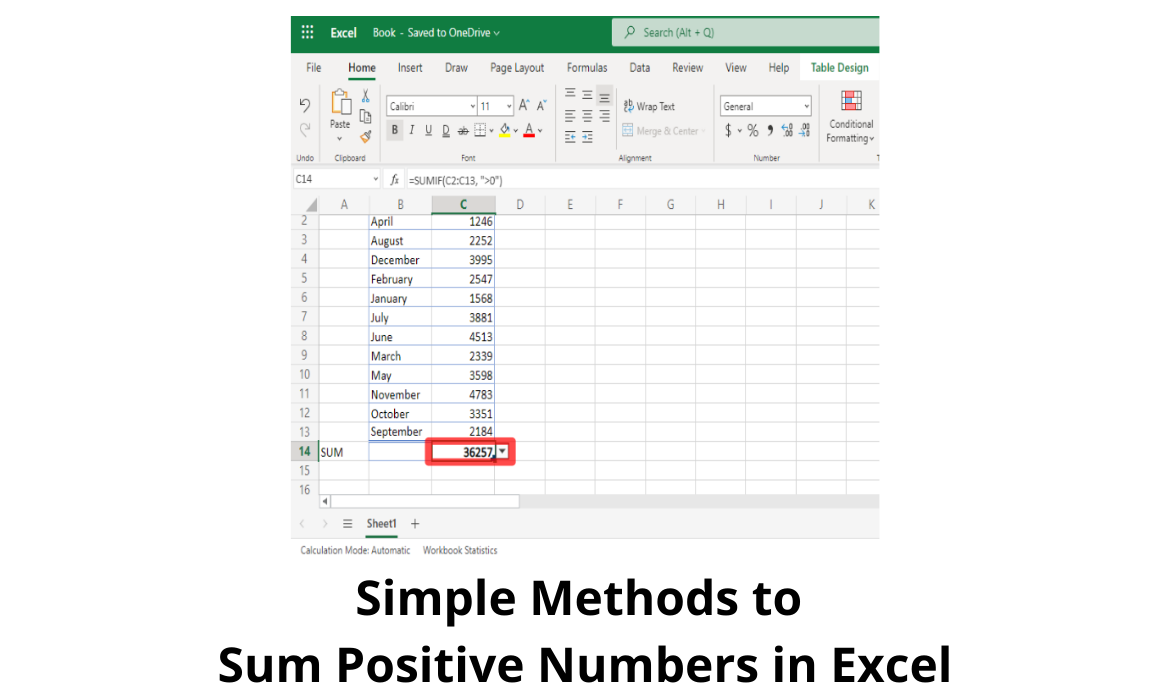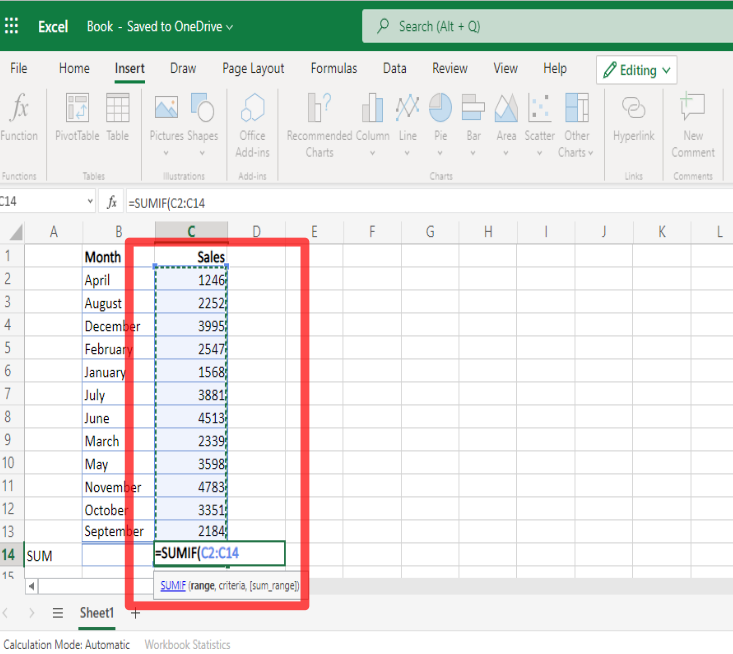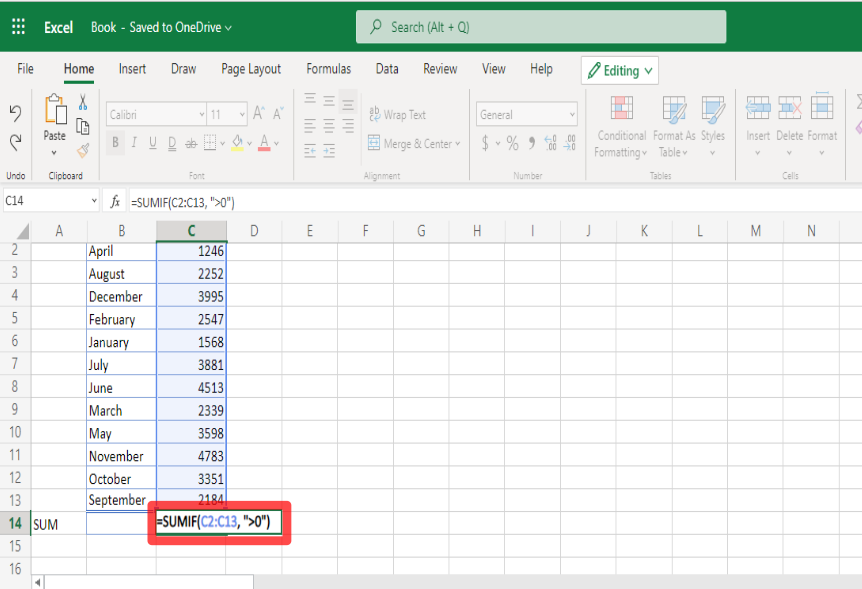# Sum Positive Numbers in Excel using These Easy MethodsAt some point, it may become necessary to add up a large amount of data within a short amount of time.

This is where getting the sum of values in Excel could become critical.

While summing positive numbers up can be done manually, it can be done a lot quicker and more efficiently in Excel.

## Here are Easy ways to Sum (or ADD) Positive Numbers/Values in Excel:

There happen to be a few effective methods that can be used to add up positive values in Excel.

Here, we will work through a couple of techniques that can be effective for getting the sum of a set of numbers within a cell range.

Let’s begin by having a look at the first solution:

### Apply the SUMIF Function

SUMIF is a function that is commonly used for summing up contents of a cell range.

With SUMIF, you can specify the kind of numbers which you would like summed, while leaving other types of numbers out of this process, if desired.

For example, SUMIF happens to be a highly effective tool for adding up positive numbers in Excel worksheets.

You might soon find that using SUMIF for this purpose can be easy and that it can help you to create the sums which you are looking for.

To begin with this technique, follow these steps:

1. Locate the cell that you desire the sum to appear on the spreadsheet (for instance, in the cell immediately beneath the column which contains the values to be summed).
2. Within that cell, type =SUMIF(3. Click and drag to highlight and select the cells which you desire to be summed up. The example range in this tutorial goes from C2 to C14 to show you how it works. Make sure to select the specific range of cells that you want to sum.4. The range of the cells that you selected should now be visible next to the left parenthesis of the SUMIF function in the cell that you typed the function in, as well as within the formula bar.5. If you would like to sum only positive numbers, you will have to specify a condition within the SUMIF function. The condition that we will use for this method is >0 which will set it to numbers which are above 0, or positive numbers only.

To set the condition, go to the cell that contains the SUMIF formula, write a comma along with a set of quotation marks after the cell range, specify and type the condition within the quotation marks, then type a closing or right parenthesis to close it off.

Example: =SUMIF(C2: C14, “>0”)1. Click on Return or Enter to get the result.

When SUMIF has been used as described in the steps above, you should be able to sum the positive numbers on your spreadsheet.

Note: Using the condition “<0” will allow you to sum up any negative numbers if you desire to.

In order to sum up the negative values, you would follow the same process, except insert  the “<0” condition in place of “>0”.### Summing Positive Numbers with VBA Code

VBA means Visual Basic for Applications.

VBA is a programming language that is commonly used to complete tasks in Excel.

VBA provides you with a way to get things done by applying code.

This tool can also be used to sum up positive numbers in Excel.

If you don’t mind using some code to add up the positive numbers, then this technique can get the job done.

To put this method to use:

1. Go to ‘Developer’ in the ribbon menu, then click on the Visual Basic option. The window for VBA should now come up.
2. Press and select ‘Insert’, then select ‘Module’ from the submenu that opens.
3. Copy the VBA code that adds up positive numbers in Excel. You can use the code below:
```Sub Sum_only_positive_numbers()
Dim ws As Worksheet
Dim rng As Range
Dim result As Range
Set ws = Application.ActiveSheet
Set rng = Application.Selection
Set result = Application.InputBox(_
Title:=” Get Location for Displaying Result”, _
Prompt:=”Select the cell where you want the result to appear”, _
Type:=8)
Result.Value = Application.WorksheetFunction.SumIf(rng, “>0”)
End Sub```
1. Next, paste the code. You can now close the VBA window if you wish to, as the code is ready to be used.
2. Go back to the spreadsheet and click and drag to select the cells which have the positive numbers that you want to sum.
3. Go to ‘Developer’ on the ribbon menu again, then press and select ‘Macros’. A dialog box should then pop up.
4. From the Macros dialog box, press and click on ‘Sum_only_positive_numbers’ from the group of macros that you see, then press OK.
5. The code should start running. When a prompt comes up and tells you to select a cell location for the sum, click on the cell where you want it to appear, then click on OK.

After you have used the VBA code with this method, the numbers of the selected cell range should be summed with the result shown in the cell that you specified.

Note: If you would like to add up any negative values of a specific set of cells instead of positive values, just change the condition from “>0” to “<0” within the VBA code.

## Achieving the Summed Result

We have looked over how to sum positive numbers in Excel.

Feel free to go through any method above until you have gotten the desired positive numbers summed.

Denise is a Financial Controller with experience in Office Software (Excel & Word), Accounting, Bookkeeping, Business Incorporation/LLC, Taxes and IRS Issues and more. She Graduated from Portland State University in Business Management and went onto Seattle University to complete her Graduate (Masters) Degree in Organizational Systems Renewal. She contributes to Computing.net with her knowledge of Business Software, in particularly Microsoft Excel and Google Sheets.